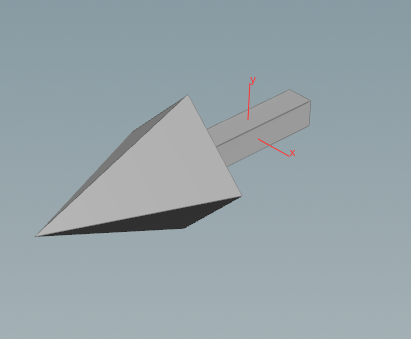## Recommended Posts

Hi all i'm going over some concepts such as rotation and the orient attribute by doing some simple examples.

I would appreciate if someone could clear up the confusion I'm having or point out where I've gone wrong

Simply put - I have an Arrowthat i'm copying to points that are on

1) a line with @N = {0,0,1};

2) a noise deformed grid

The line is pointing in the Z axis before it is passed on to the copy to points sop.

Here is what i'm trying to understand:

I have an point wrangle on the Line and the Grid with the following where @up is set to {0,1,0} and the Angle channel is animated using @Frame;

```v@up = chv("up_vector");

vector nCrossUp = normalize(cross(@N,@up)); // Commenting out this line and the one below gives weird rotations
v@up = normalize(cross(nCrossUp,@N));

matrix3 m = maketransform(@N, v@up);

vector axis = normalize(@N);

p@orient = quaternion(m);```

This results in the arrows rotating on their @N axis on both the line and grid.

My understanding is that:

We have two approaches when dealing with orientations:

1) Using the cross product of the N and the Up and then crossing the resultant vector again by the @N to get proper @up vectors.

2) We can use the @orient to stabilise the rotation when the template points are deforming.

Furthermore, in the line example above, the Normals of the template points are pointing in the Z-Axis. When I change the normals to be pointing in @N = {0,1,0} it breaks (because the N and the Y are both in the same direction?) How would I go about doing this properly and is there a need to use the cross product approach when using the @orient method?

I have attached the hip file to this post if you want to look at what I've done.

orient_Arrows_v01.hiplc

## Create an account

Register a new account